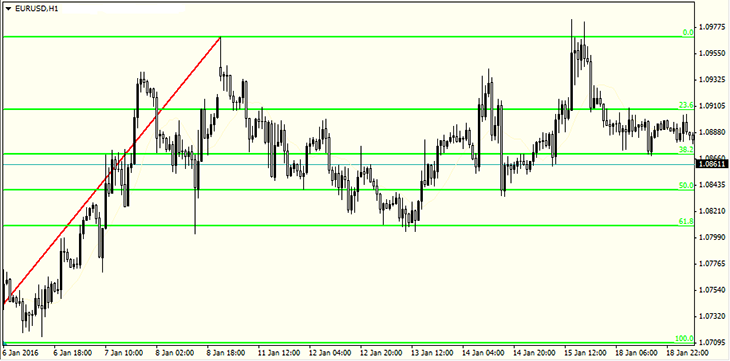Fibonacci Retracement Levels | How to Use Fibonacci Retracement | What is Fibonacci Retracement | IFCM

# Fibonacci Retracement Levels

## What is Fibonacci Retracement?

Fibonacci Retracement is an important and effective tool of technical analysis for determining the possible levels of trend reversal. By taking Fibonacci Retracement levels into account the trader can determine the strong support and resistance levels, as well as the possible targets of correction and continuation trends.

which account to choose

## Fibonacci Numbers

The Fibonacci sequence of numbers became popular in Europe thanks to an Italian mathematician Leonardo Pisano (Fibonacci), although, this sequence had been known in the East long before him. "The sequence presents a series of numbers, where each subsequent number is the sum of the previous two:

0, 1, 1, 2, 3, 5, 8, 13, 21, 34, 55, 89, 144, 233, 377……

The Fibonacci sequence of numbers extends to infinity and contains many unique mathematical properties.

• If any of the numbers of this sequence is divided into the following number, then the result will be approximately 0.618 (13/21=0.619, 21/34=0.617, 34/55=0.618).
• If any of the numbers of this sequence is divided into the previous number, then the result will be approximately 1.618 (21/13=1.6153, 34/21=1.6190, 55/34=1.6176).

Last sequence was used in the financial market by the famous trader Ralph Elliott. In his theory of waves, Ralph noticed that the ratio of the height of the next wave to the previous one is approximately equal to 1,618.

## Start earning now in giant market

Forex 5.1\$ trillion daily

## How to Use Fibonacci Retracement

Practically, in any platform there is an instrument “Fibonacci Lines” with the help of which one can create correction levels - 0%, 23,6%, 38.2%, 50%, 61.8%, 76,4% and 100%. The Fibonacci lines also allow to determine the target of correction in case the trend continues – it is 161.8%, 261.8% and 423.6%. In case of an uptrend, the lines should be stretched from bottom to top, and in case of a downtrend – from top to bottom.## How to Trade with Fibonacci

Trading on Fibonacci levels involves two ways.

• The opening of a long positions in case of a rebound from the support line, when the market goes up.
• The opening of a short position in case of a rebound from resistance level, when the market goes down.

Since Fibonacci levels are supposed to be support and resistance levels then trading based on these levels is conducted in the same way as in trading strategies based on support and resistance levels.

Build a Fibonacci Retracement Levels in the terminal
Once opened Demo you will be supplied with educational materials and online support in your own language

### How to use Fibonacci Retracement Levels in trading platformYou can study CFD trading more thoroughly and see CFD trading examples in the section How To Trade CFDs